##### Actions

Letbe a prime number; an-adic sheaf on a schemeis a projective system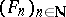of étale Abelian sheavessuch that, for all, the transfer homomorphisms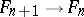are equivalent to the canonical morphism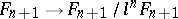. An-adic sheafis said to be constructible (respectively, locally constant) if all sheaves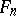are constructible (locally constant) étale sheaves. There exists a natural equivalence of the category of locally constant constructible sheaves on a connected schemeand the category of modules of finite type over the ring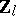of integral-adic numbers which are continuously acted upon from the left by the fundamental group of the scheme. This proves that locally constant constructible sheaves are abstract analogues of systems of local coefficients in topology. Examples of constructible-adic sheaves include the sheaf, and the Tate sheaves(where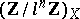is the constant sheaf onassociated with the group, whileis the sheaf of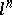-th power roots of unity on). Ifis an Abelian scheme over, then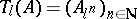(where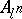is the kernel of multiplication byin) forms a locally constant constructible-adic sheaf on, called the Tate module of.
Letbe a scheme over a field, letbe the scheme obtained fromby changing the base fromto the separable closure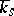of the field, and letbe an-adic sheaf on; the étale cohomology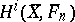then defines a projective system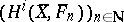of-modules. The projective limit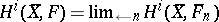is naturally equipped with the structure of a-module on which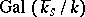acts continuously with respect to the-adic topology. It is called the-th-adic cohomology of the sheafon. If, the usual notation is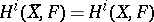. The fundamental theorems in étale cohomology apply to-adic cohomology of constructible-adic sheaves. If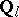is the field of rational-adic numbers, then the-spacesare called the rational-adic cohomology of the scheme. Their dimensions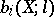(if defined) are called the-th Betti numbers of. For complete-schemes the numbersare defined and are independent of(). Ifis an algebraically closed field of characteristicand if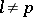, then the assignment of the spaces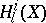to a smooth complete-variety defines a Weil cohomology. Ifis the field of complex numbers, the comparison theorem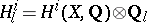is valid.